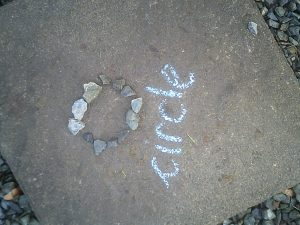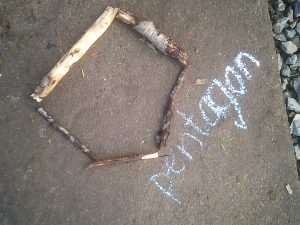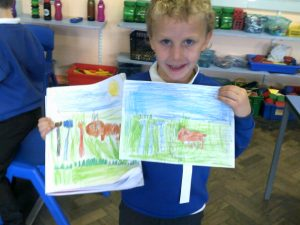## Maths Homework for Monday 23rd November

This weeks Maths Challenge is for your child to learn their number bonds.  You will not need to email or upload evidence this week of your child learning these bonds as we will be assessing them on their knowledge on Monday 23rd November.

Many Thanks

Triongl Group –  Learn your number bonds (pairs) to 5.

0 + 5 = 5

1 + 4 = 5

2 + 3 = 5

3 + 2 = 5

4 + 1 = 5

5 + 0 = 5

Sgwar Group –  Learn your number bonds (pairs) to 10.

0 + 10 = 10

1 + 9 = 10

2 + 8 = 10

3 + 7 = 10

4 + 6 = 10

5 + 5 = 10

6 + 4 = 10

7 + 3 = 10

8 + 2 = 10

9 + 1 = 10

10 + 0 = 10

Pentagon and Cylch Group –  Learn your number bonds (pairs) to 20.

0 + 20 = 20

1 + 19 = 20

2 + 18 = 20

3 + 17 = 20

4 + 16 = 20

5 + 15 = 20

6 + 14 = 20

7 + 13 = 20

8 + 12 = 20

9 + 11 = 20

10 + 0 = 20

11 + 9 = 20

12 + 8 = 20

13 + 7 = 20

14 + 6 = 20

15 + 5 = 20

16 + 4 = 20

17 + 3 = 20

18 + 2 = 20

19 + 1 = 20

20 + 0 = 20

## Jonah and the Whale

The children have enjoyed reading the story of ‘Jonah and the Whale’.  They then used their reading skills to read instructions on how to make a whale of their choice.

## Maths Enrichment Day

The children have enjoyed working outside this morning completing a variety of Maths activities as part of of Maths Enrichment Day.

## Eryr and Gwennol Maths Homework

Task for children in groups Triongl/Sgwar

Use natural objects to make the following shapes….triangles, circles, squares and rectangles.

Here is an example:Task for children in groups Pentagon/CylchUse natural objects to make the following shapes….pentagons, hexagons and octagons.  They can be regular or irregular.

Here is an example:Many thanks

## Diwali

The children have been finding out about the Hindu festival of Diwali.  Diwali is the Hindu festival of light.  The children used their reading skills to read and follow instructions to make diva lamps, lanterns, elephant decorations and Rangoli decorations.

## Healthy Eating

The children have been busy looking at what foods make a ‘balanced diet’.  They worked in groups to create a healthy lunchbox.

## We Will Remember!

With Remembrance Day approaching, the children have enjoyed looking at the artwork ‘Field of Poppies’ by Vincent Van Gogh and creating their own artwork.  The children have used a variety of materials to create their own poppy field artwork.

The children are looking forward to taking their artwork home to display as way of commemorating Remembrance Day.

## Technology Day

Year 1 and Year 2 pupils have enjoyed designing and making their own pop-up moving story books in groups.Digital Camera